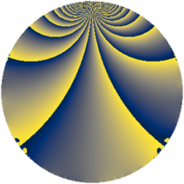# Properties

 Label 585.2.btLevel $585$ Weight $2$ Character orbit 585.bt Rep. character $\chi_{585}(376,\cdot)$ Character field $\Q(\zeta_{6})$ Dimension $112$ Newform subspaces $2$ Sturm bound $168$ Trace bound $1$

# Related objects

## Defining parameters

 Level: $$N$$ $$=$$ $$585 = 3^{2} \cdot 5 \cdot 13$$ Weight: $$k$$ $$=$$ $$2$$ Character orbit: $$[\chi]$$ $$=$$ 585.bt (of order $$6$$ and degree $$2$$) Character conductor: $$\operatorname{cond}(\chi)$$ $$=$$ $$117$$ Character field: $$\Q(\zeta_{6})$$ Newform subspaces: $$2$$ Sturm bound: $$168$$ Trace bound: $$1$$ Distinguishing $$T_p$$: $$2$$

## Dimensions

The following table gives the dimensions of various subspaces of $$M_{2}(585, [\chi])$$.

Total New Old
Modular forms 176 112 64
Cusp forms 160 112 48
Eisenstein series 16 0 16

## Trace form

 $$112 q + 56 q^{4} - 8 q^{9} + O(q^{10})$$ $$112 q + 56 q^{4} - 8 q^{9} - 24 q^{12} + 2 q^{13} - 56 q^{16} + 32 q^{17} - 24 q^{23} + 56 q^{25} + 24 q^{26} + 48 q^{27} - 20 q^{29} + 32 q^{30} - 32 q^{35} - 52 q^{36} - 44 q^{38} + 44 q^{39} - 28 q^{42} - 16 q^{43} - 68 q^{48} + 52 q^{49} + 28 q^{51} + 10 q^{52} - 80 q^{53} + 40 q^{56} + 28 q^{61} + 88 q^{62} - 184 q^{64} - 2 q^{65} - 144 q^{66} - 4 q^{68} - 140 q^{69} + 40 q^{74} + 16 q^{77} - 58 q^{78} + 16 q^{79} + 16 q^{81} + 72 q^{82} + 60 q^{87} + 20 q^{90} + 180 q^{92} - 36 q^{94} - 40 q^{95} + O(q^{100})$$

## Decomposition of $$S_{2}^{\mathrm{new}}(585, [\chi])$$ into newform subspaces

Label Dim $A$ Field CM Traces $q$-expansion
$a_{2}$ $a_{3}$ $a_{5}$ $a_{7}$
585.2.bt.a $4$ $4.671$ $$\Q(\zeta_{12})$$ None $$0$$ $$6$$ $$0$$ $$0$$ $$q+2\zeta_{12}q^{2}+(2-\zeta_{12}^{2})q^{3}+2\zeta_{12}^{2}q^{4}+\cdots$$
585.2.bt.b $108$ $4.671$ None $$0$$ $$-6$$ $$0$$ $$0$$

## Decomposition of $$S_{2}^{\mathrm{old}}(585, [\chi])$$ into lower level spaces

$$S_{2}^{\mathrm{old}}(585, [\chi]) \cong$$ $$S_{2}^{\mathrm{new}}(117, [\chi])$$$$^{\oplus 2}$$